Control Index

Control System Block Manipulation

The table below relates the control block symbols to the control equation and also illustrates how changes in the control block arrangements can be changed whilst retaining the original control function.

 Block Operation Original Arrangement Modified Arrangement Equation Blocks In Series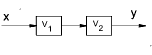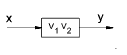y =  V1.V2.x Blocks in Parallel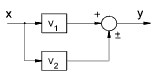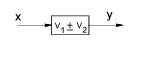y  =  (V1 ± V2) . x Blocks in Feedback Loop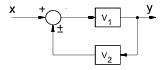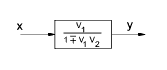y  =  V1( x ± y . V2) or    y =  [ V1 / (1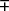V1.V2 ) ] .x Moving a Summing Block Ahead of an element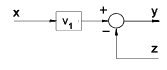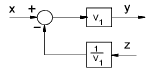y  =  V1.x  -  z Moving a Summing Block beyond of an element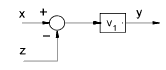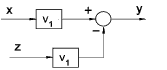y  =  V1.(x  - z) Moving an take-off point ahead of an element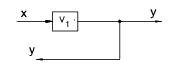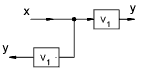y  =  V1. x Moving an take-off point beyond an element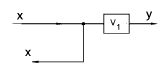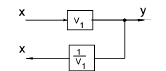y  =  V1. x Removing an element from a forward path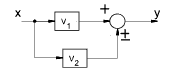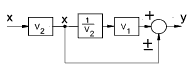y =  x. ( V1 ±  V2 ) Inserting an element in a forward path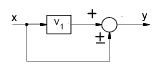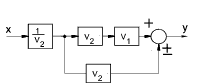y =  V1. x  ±  x Removing an element from a feedback path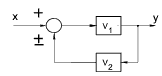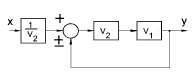y = V1( x ± y . V2) or    y =  [ V1 / (1V1.V2 ) ] .x Inserting an element in a feedback path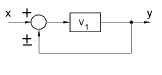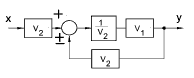y  =  V1( x ± y ) or    y  =  [ V1 / (1V1) ] .x Rearrangement of summing points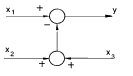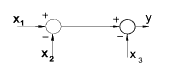y  =  x1  -   x2  -   x3 Interchange of summing points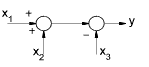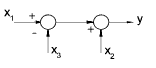y  =  x1   +   x2   -   x3 Moving a Take-off point ahead of a summing point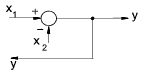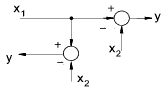y  =  x1  -   x2 Moving a Take-off point beyond a summing point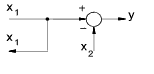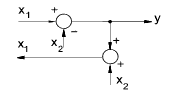y  =  x1   -   x2 x1   =   y  +   x2

 Sites & Links For Control Information Principles of Control Systems..Notes On control system diagrams pdhcenter.com/courses..Automatic Control Systems - Part I:Block Diagrams and Transfer Functions 8- Feedback Control Systems..Detailed set of notes

Control Index